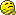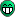# Creating TF1 from TGraph

Dear rooters,
i’ve been looking on the forum for this but afaik there is not a complete answer to this problem.

It would be nice to have an easy way to create a TF1 from the linear interpolation of a TGraph since there are so many operations you can’t do with the TGraph. For example if you want to find the x points at which the graph intersect a certain constant y value, you have to create an ad hoc root finding algorithm that gets the graph as a parameter.

Is there an easy way to create this TF1? I’m aware of the function TGraph::Eval , however if you write:

TGraph *graph = new TGraph(n,x,y);
TF1 f = new TF1(“f”,"(graph->Eval(x))",x0,x1);
f->SetParameter(0,1);

it does not work. I believe that “graph->Eval(x)” is a bad formula expression and TF1 cannot use it.

I’m sure there is a simple solution to this, nonetheless i wasn’t able to find a macro that helps me with this issue.

Thanks

Matteo

What you request is not possible in general because 2 x values of a TGraph may have the same y value.
However, it is easy to solve your problem by creating a TF1 with a C-style function as shown in the following code snippet.

[code]TGraph *gr;
Double myfunc(Double_t *x, Double_t *) { return gr->Eval(x[o]);}

void mycode() {
gr = new TGraph(…

TF1 *f1 = new TF1(“f1”,myfunc,xmin,xmax,0);
}
[/code]
Rene

Thank you very much!

Dear all,

I am having the same problem mentioned above (I would like to create a TF1 from a TGraph or a TSpline3 if it is possible).
However, the solution proposed in this topic is not working for me.

[code]
/* c++ includes */
#include
#include <stdio.h>
#include

/* root includes */
#include “TROOT.h”
#include “TRandom3.h”
#include “TTree.h”
#include “TProfile.h”
#include <TMath.h>
#include <TString.h>
#include <TFile.h>
#include <TCanvas.h>
#include <TGraph.h>
#include <TAxis.h>
#include <TF1.h>
#include
#include “TVectorD.h”
#include “TSystem.h”
#include “TLatex.h”
#include <TSpline.h>
#include “TMultiGraph.h”
#include “TRint.h”

using namespace std;

// TGraph to TF1
TGraph *g1;
double myfunc(double *xx, double *)
{
return g1->Eval(xx);
}

// Main function

int main(int argc, char *argv[])
{
int fargc = 1;
TRint *rint = new TRint(“Test”, &fargc, argv);

``````// Opening data. Here energy and PAI cross section relative to Rutherford cross section is provided
TTree *MyTree = new TTree("MyTree","MyTree");
Double_t energy, crossSection;

// Probability of energy deposition
Int_t nn = MyTree->GetEntries(); // number of entries on .txt file
const Int_t n = nn;
TVectorD ene(n), Pn1(n), sigma(n), Sigma(n), SSigma(n);
TVectorD dSigma_dE(n), dSigmaRuth_dE(n), dSigmaRuth(n);

const Double_t beta_lorentz = 0.97;
const Double_t betagamma = beta_lorentz/sqrt(1 - pow(beta_lorentz,2));
const Double_t mc2 = 0.510998928*pow(10,6);
const Double_t Emax = 2*mc2*pow(betagamma,2);
const Double_t kr = 2.54955*pow(10,-19); // constant used in Rutherford cross-section (eV.cm2)

for (int i=0; i<n; i++) // loop on all values of energy
{
MyTree->GetEntry(i);
ene(i) = energy;
sigma(i) = crossSection;

// Rutherford cross-section
dSigmaRuth_dE(i) = kr/pow(beta_lorentz*ene(i),2)*(1 - pow(beta_lorentz,2)*ene(i)/Emax);

// Converting inital cross section
Sigma(i) = sigma(i)*dSigmaRuth_dE(i);
}

TCanvas *c1 = new TCanvas("c1","c1",800,600);
c1->cd();
TGraph *g1 = new TGraph(ene,Sigma);
TSpline3 *s1 = new TSpline3("interpolation1",g1);
s1->Draw();
Double_t emin = 9.203623;
Double_t emax = 94867.336;
TF1 *f1 = new TF1("f1",myfunc,emin,emax);
Double_t area = f1->Integral(emin,emax);
cout << " Area: " << area << endl;
rint->Run(kTRUE);
}
[/code]
``````

And I am having the following message when the line “double newsum = f1->Integral(Emin,Emax);” is not commented:

*** Break *** segmentation violation
Generating stack trace…
0x00000001111b0c73 in TF1_EvalWrapper::DoEval(double) const (in libHist.so) + 35
0x0000000112142ff2 in ROOT::Math::GaussIntegrator::DoIntegral(double, double, ROOT::Math::IBaseFunctionOneDim const*) (in libMathCore.so) + 482
0x00000001111abcf8 in TF1::Integral(double, double, double const*, double) (in libHist.so) + 200
0x000000010f39a060 in main (in paiBichselv2) + 3520
0x00007fffeb7f8255 in start (in libdyld.dylib) + 1

Thanks for any help!
Best,

Diego
data-Bichsel.txt (2.91 KB)

I have converted your program into a script which can be run interactively in root.

``````// TGraph to TF1
TGraph *g1;
double myfunc(double *xx, double *)
{
return g1->Eval(xx);
}

// Main function

void diego()
{
// Opening data. Here energy and PAI cross section relative to Rutherford cross section is provided
TTree *MyTree = new TTree("MyTree","MyTree");
Double_t energy, crossSection;

// Probability of energy deposition
Int_t nn = MyTree->GetEntries(); // number of entries on .txt file
const Int_t n = nn;
TVectorD ene(n), Pn1(n), sigma(n), Sigma(n), SSigma(n);
TVectorD dSigma_dE(n), dSigmaRuth_dE(n), dSigmaRuth(n);

const Double_t beta_lorentz = 0.97;
const Double_t betagamma = beta_lorentz/sqrt(1 - pow(beta_lorentz,2));
const Double_t mc2 = 0.510998928*pow(10,6);
const Double_t Emax = 2*mc2*pow(betagamma,2);
const Double_t kr = 2.54955*pow(10,-19); // constant used in Rutherford cross-section (eV.cm2)

for (int i=0; i<n; i++) {
MyTree->GetEntry(i);
ene(i) = energy;
sigma(i) = crossSection;

// Rutherford cross-section
dSigmaRuth_dE(i) = kr/pow(beta_lorentz*ene(i),2)*(1 - pow(beta_lorentz,2)*ene(i)/Emax);

// Converting inital cross section
Sigma(i) = sigma(i)*dSigmaRuth_dE(i);
}

TCanvas *c1 = new TCanvas("c1","c1",800,600);
c1->cd();
g1 = new TGraph(ene,Sigma);
TSpline3 *s1 = new TSpline3("interpolation1",g1);
s1->Draw();
Double_t emin = 9.203623;
Double_t emax = 94867.336;
TF1 *f1 = new TF1("f1",myfunc,emin,emax);
Double_t area = f1->Integral(emin,emax);
cout << " Area: " << area << endl;
}``````

it gives:

``````\$ root diego.C
-----------------------------------------------------------------
| Welcome to ROOT 6.09/01                     http://root.cern.ch |
|                                    (c) 1995-2016, The ROOT Team |
| Built for macosx64                                              |
| Try '.help', '.demo', '.license', '.credits', '.quit'/'.q'      |
-----------------------------------------------------------------

root 
Processing diego.C...
Area: 1.84579e-20
root  ``````

and the attached plot

Dear Couet,

Thanks for your prompt response. Indeed, writing the code in that way, it is working. However, There is no agreement about the integral value. You have found Area = 1.84579e-20. I have found, using root 5.34/36, Area = 3.35738e-21 doing exactly the same procedure you did. Would the different version change anything? In addition, I could compare these results with an integral I have implemented by myself using trapezoidal rule, and I got Area = 5.44068e-20. I believe the latter is the right one because I could compare with a reliable source. Any idea about this disagreement?

Cheers,

Diego

It seems f1->Integral(emin,emax); has a different behavior from a version to an other. I will ask the expert.

HI,

Yes this is true. In ROOT 6 a new algorithm from the libMathMore library is used, when it is available. This new algorithms should work in general better especially in case of peaked functions, but of course there could be some cases where it does not work and the other does.

To use the old algorithm in ROOT 6, do before calling TF1::Integral

ROOT::Math::IntegratorOneDimOptions::SetDefaultIntegrator(“Gauss”);

Lorenzo

Hello,

Yes, indeed ROOT 6 gives a more accurate result. However, I still have a factor of 3 compared to the true integral. Probably the algorithms provided by ROOT are not adapted to my problem.

Thanks anyway!

Diego

Why are you still complainingYou now have three different integral values, so you can choose the one you like the mostUsing “ROOT::Math::IntegratorOneDimOptions::SetDefaultIntegrator(…);”, you could try all different available ROOT’s “1-dim integrators”: “Gauss”, “GaussLegendre”, “Adaptive”, “AdaptiveSingular” and “NonAdaptive”.You will then have six different integral values to choose fromBTW. ROOT’s “integrators” are well known to misbehave in all cases when the function “changes rapidly” or if there are “narrow / sharp peaks” inside. I think that, in vast majority of cases like yours, people “blindly” use the returned “integral” without noticing that it is wrong. What’s even worse, “integrals” are quietly calculated and used internally by some methods in various classes (users may not even realize that this happens).